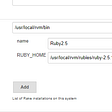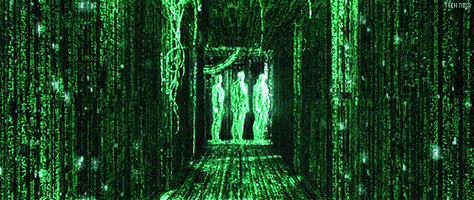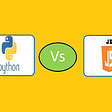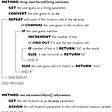# Longest Common Subsequence — Day 51(Python)

Today’s question is a famous question that is solved using Dynamic Programming. You will find many other questions that are variations of this question. Let us jump into the question without further ado. We will be solving this problem in a 3 part series. Today, we will be solving this problem using recursion.

1143. Longest Common Subsequence

Given two strings `text1` and `text2`, return the length of their longest common subsequence.

A subsequence of a string is a new string generated from the original string with some characters(can be none) deleted without changing the relative order of the remaining characters. (eg, “ace” is a subsequence of “abcde” while “aec” is not). A common subsequence of two strings is a subsequence that is common to both strings.

If there is no common subsequence, return 0.

Example 1:

`Input: text1 = "abcde", text2 = "ace" Output: 3  Explanation: The longest common subsequence is "ace" and its length is 3.`

Example 2:

`Input: text1 = "abc", text2 = "abc"Output: 3Explanation: The longest common subsequence is "abc" and its length is 3.`

Example 3:

`Input: text1 = "abc", text2 = "def"Output: 0Explanation: There is no such common subsequence, so the result is 0.`

Constraints:

• `1 <= text1.length <= 1000`
• `1 <= text2.length <= 1000`
• The input strings consist of lowercase English characters only.

The question wants to find the length of the longest common subsequence. What does a subsequence mean?

According to Wikipedia

A subsequence is a sequence that can be derived from another sequence by deleting some or no elements without changing the order of the remaining elements.

Let us take a few examples.

Now that we understand what a subsequence is, let us move to the gist of the problem. According to the problem, we need to find the longest subsequence between both the given input strings. The characters from both the strings are compared each time, in case they match, smaller strings are compared next. If the characters do not match, characters are eliminated one by one. It sounds confusing. Let us break it down.

What is the input to the recursive function? We would have two strings which are the input to the recursive function. Each time the recursive function is called, the length of the input strings reduces.

Before thinking of the recursive function, we need to identify the base condition. What is the smallest, valid input for the function? If any one of the string is empty, we will return 0. Well, if any of the strings are null, there is nothing in common, we return 0.

`if len(text1) == 0 or len(text2) == 0:   return 0`

We have understood the base condition. Now let us build the recursive function.

We start from the end of both the strings; there are two possibilities here. The characters in the current index are the same or they are not. If they are the same, we increment the counter, and then call the recursive function again, this time the size of the input string is reduced by one, removing character at the end of both the string. If the characters are not the same, we again have two choices. Either remove the character at the end of string 1 or remove the character at the end of string 2. Why are we doing it?

Let us understand with an example.

In the above example, if we notice, in the second pass, the character at the second last position of string 1 and the last character of string 2 is equal. We have two choices, either we reduce the size of string 1 or we reduce the size of string 2. Since we need to find the longest common subsequence, we will return the maximum from both the results.

`if text1[-1] == text2[-1]:   return 1+(self.longestCommonSubsequence(text1[:-1],text2[:-1]))else:   return max(self.longestCommonSubsequence(text1[:-1],text2), self.longestCommonSubsequence(text1,text2[:-1]))`

Let us look at the entire code snippet.

`class FindLongest:    def longestCommonSubsequence(self, text1: str, text2: str) -> int:        if len(text1) == 0 or len(text2) == 0:            return 0        if text1[-1] == text2[-1]:            return 1+(self.longestCommonSubsequence(text1[:-1],text2[:-1]))        else:            return max(self.longestCommonSubsequence(text1[:-1],text2), self.longestCommonSubsequence(text1,text2[:-1]))`

Complexity analysis.

Time Complexity

At each point, we have two choices, either to decrement the size of string 1 or string 2. Hence the time complexity is O(2^N*M), where N is the size of string 1 and M is the size of string 2.

Space Complexity.

The space complexity of this code is O(2^N*M).

--

--

--

## More from Annamariya Tharayil

Software Engineer. Find me @ www.linkedin.com/in/annamariya-jt

Love podcasts or audiobooks? Learn on the go with our new app.

## CI/CD of Ruby On Rails using Jenkins## Being “pedantic” about the code you write — The Flutter way.## Merging the Enemy functionality## Enter the Internet## Schema.org Just Released New Restaurant Menu Markup Heres How to Get Started## Remove the background from your photos with Python## Python is a high-level interpreted programming language with dynamic semantics and object-oriented…## Getting started with SQL! (Part 1)## Annamariya Tharayil

Software Engineer. Find me @ www.linkedin.com/in/annamariya-jt

## Is java compiler-based or Interpreter based ?## From Excel Sheet to Postman Request## TIL 0624 Head First Java Ch.5 — Extra-Strength Methods## Pyscript -Run Python code in Html just like Javascript